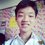# A Pair Of Elementary Logarithmic Integrals

Here are two beautiful elementary logarithmic integrals which I came across recently: $\textrm{i) Let} \ m, n \ \textrm{be natural numbers. Prove that} \int_{0}^1 x^m \log^n (x) \, dx=(-1)^n\frac{n!}{(m+1)^{n+1}}.$ $\textrm{ii) Prove that} \int_{0}^a x^m \log^n (x) \, dx=a^{m+1} \sum_{k=0}^n (-1)^k\binom n k \frac{k!}{(m+1)^{k+1}} \times \log^{n-k}(a) , a>0.$

I'll present my own solution to i) and ii).

Solution i)

Let $I(m,n)$ denote the integral, $\displaystyle \int_{0}^1 x^m \log^n (x) \, dx$, by using IBP, we have

\begin{aligned} I(m,n)&=\left .\frac{1}{m+1} \log^n (x) x^{m+1}\right|_{0}^{1}-\frac{m}{m+1}\int_{0}^1 x^m \log^{n-1} (x) \, dx \\ &=-\frac{n}{m+1}I(m,n-1) \\ &=(-1)^2\frac{n(n-1)}{(m+1)^2} I(m,n-2) \\ &=\ldots \\ &=(-1)^{n}\frac{n!}{(m+1)^n} I(m,0) \\ &=(-1)^{n}\frac{n!}{(m+1)^n} \int_{0}^1 x^m \, dx \\ &=(-1)^n\frac{n!}{(m+1)^{n+1}}. \ \blacksquare \end{aligned}

Solution ii)

Let $I(m,n)$ denote the integral, $\displaystyle \int_{0}^a x^m \log^n (x) \, dx$, by using IBP, we have

\begin{aligned} I(m,n)&=\left .\frac{1}{m+1} \log^n (x) x^{m+1}\right|_{0}^{a}-\frac{n}{m+1}\int_{0}^{a} x^m \log^{n-1} (x) \, dx \\ &=(-1)^0 \binom n 0 \frac{0!}{(m+1)^{0+1}} \log^{n-0} (x) a^{m+1}-\frac{1}{m+1} \binom n 1 I(m,n-1) \\ &=a^{m+1}(-1)^0\binom n 0 \frac{0!}{(m+1)^{0+1}} \times \log^{n-0} (a)-\frac{1}{m+1} \binom n 1 \left(\frac{1}{m+1} \log^{n-1} (x) a^{m+1}-\frac{n-1}{m+1}I(m,n-2)\right) \\ &=a^{m+1}(-1)^0\binom n 0 \frac{0!}{(m+1)^{0+1}} \times \log^{n-0} (a)+a^{m+1} (-1)^1 \binom n 1 \frac{1!}{(m+1)^{1+1}} \times \log^{n-1} (a)+ (-1)^2 \binom n 2 \frac{2!}{(m+1)^2} I(m,n-2) \\ &=\ldots \\ &=a^{m+1}(-1)^0\binom n 0 \frac{0!}{(m+1)^{0+1}} \times \log^{n-0} (a)+a^{m+1} (-1)^1 \binom n 1 \frac{1!}{(m+1)^{1+1}} \times \log^{n-1} (a)+ a^{m+1} (-1)^2 \binom n 2 \frac{2!}{(m+1)^{2+1}} \times \log^{n-2} (a) +\ldots +(-1)^n \binom n n \frac{n!}{(m+1)^n}I(m,0) \\ &=a^{m+1}(-1)^0\binom n 0 \frac{0!}{(m+1)^{0+1}} \times \log^{n-0} (a)+a^{m+1} (-1)^1 \binom n 1 \frac{1!}{(m+1)^{1+1}} \times \log^{n-1} (a)+ a^{m+1} (-1)^2 \binom n 2 \frac{2!}{(m+1)^{2+1}} \times \log^{n-2} (a) +\ldots +a^{m+1}(-1)^n \binom n n \frac{n!}{(m+1)^{n+1}} \times \log^{n-n} (a) \\ &=a^{m+1} \sum_{k=0}^n (-1)^k\binom n k \frac{k!}{(m+1)^{k+1}} \times \log^{n-k}(a). \ \blacksquare \end{aligned}Note by ChengYiin Ong
5 months, 3 weeks ago

This discussion board is a place to discuss our Daily Challenges and the math and science related to those challenges. Explanations are more than just a solution — they should explain the steps and thinking strategies that you used to obtain the solution. Comments should further the discussion of math and science.

When posting on Brilliant:

• Use the emojis to react to an explanation, whether you're congratulating a job well done , or just really confused .
• Ask specific questions about the challenge or the steps in somebody's explanation. Well-posed questions can add a lot to the discussion, but posting "I don't understand!" doesn't help anyone.
• Try to contribute something new to the discussion, whether it is an extension, generalization or other idea related to the challenge.

MarkdownAppears as
*italics* or _italics_ italics
**bold** or __bold__ bold
- bulleted- list
• bulleted
• list
1. numbered2. list
1. numbered
2. list
Note: you must add a full line of space before and after lists for them to show up correctly
paragraph 1paragraph 2

paragraph 1

paragraph 2

[example link](https://brilliant.org)example link
> This is a quote
This is a quote
    # I indented these lines
# 4 spaces, and now they show
# up as a code block.

print "hello world"
# I indented these lines
# 4 spaces, and now they show
# up as a code block.

print "hello world"
MathAppears as
Remember to wrap math in $$ ... $$ or $ ... $ to ensure proper formatting.
2 \times 3 $2 \times 3$
2^{34} $2^{34}$
a_{i-1} $a_{i-1}$
\frac{2}{3} $\frac{2}{3}$
\sqrt{2} $\sqrt{2}$
\sum_{i=1}^3 $\sum_{i=1}^3$
\sin \theta $\sin \theta$
\boxed{123} $\boxed{123}$

Sort by:

$\int_0^1 x^m \log^n(x) dx$ taking $\log(x)=t$ and $t=-u$ $\int_{-∞}^0 e^{(m+1)t} t^n dt = \int_0^∞ (-1)^n e^{-(m+1)u} u^n du$ Taking $(m+1)u=\phi$ , then the integral becomes $\dfrac{(-1)^n }{(m+1)^{n+1}} \int_0^∞ e^{-\phi} \phi^n d\phi = \dfrac{(-1)^n \Gamma{(n+1)} }{(m+1)^{n+1}}$ $= \dfrac{n!(-1)^n }{(m+1)^{n+1}}$

- 5 months, 3 weeks ago

Here is Another way Consider $I(m)= \int_0^1 x^m dx \implies I(m) = \dfrac{1}{m+1}$ Differentiate both sides respect to $m$ for $n$ times $\dfrac{\partial^nI(m)}{\partial{m}^n}= \int_0^1 x^m \log^n(x) dx = \dfrac{(-1)^n n!}{(m+1)^{n+1}}$ A hah! :)

- 5 months, 3 weeks ago